# RATIO ANALYSIS Agenda Introduction Profitability analysis Liquidity analysis

• Slides: 28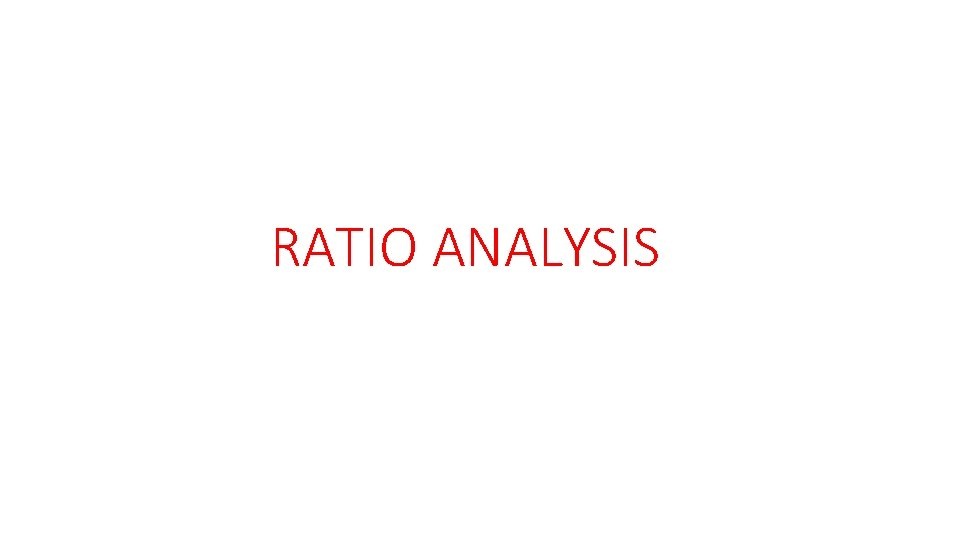RATIO ANALYSIS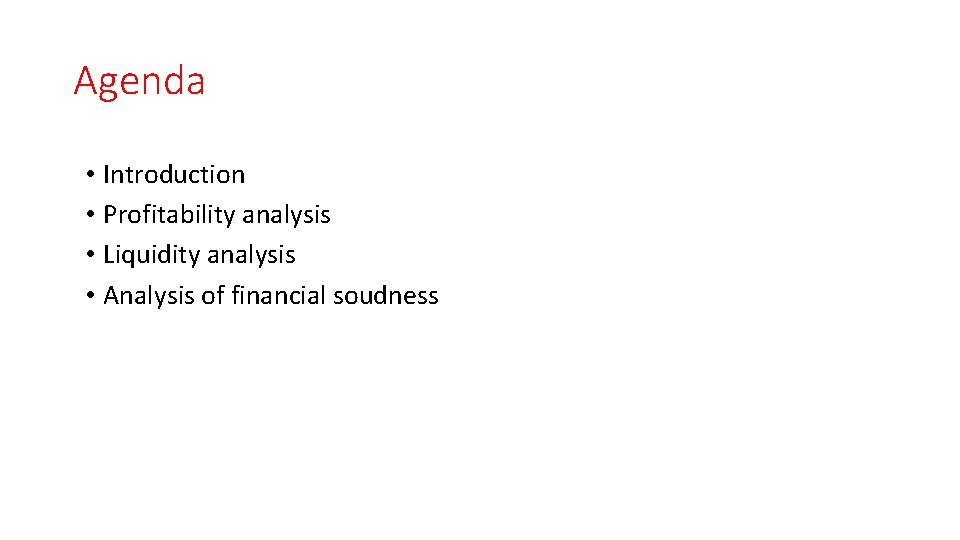Agenda • Introduction • Profitability analysis • Liquidity analysis • Analysis of financial soudness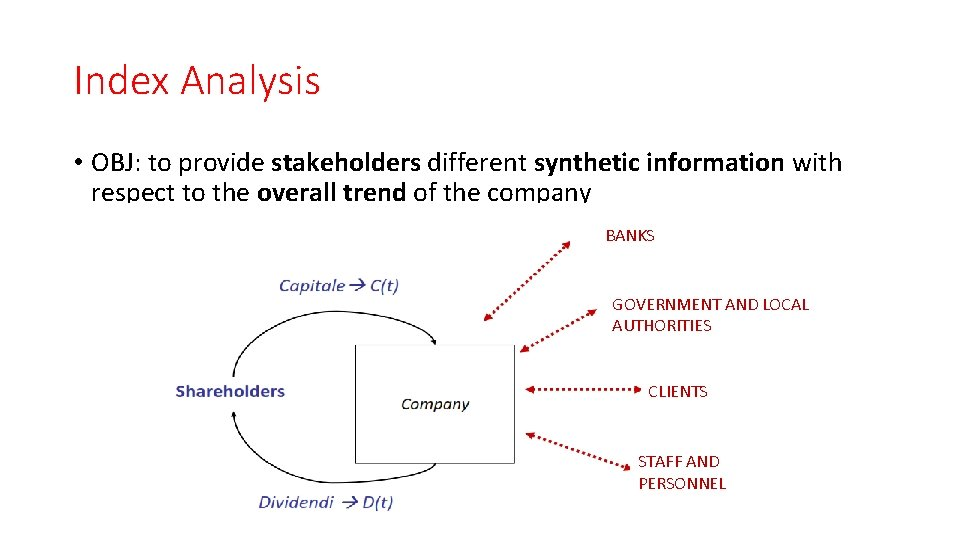Index Analysis • OBJ: to provide stakeholders different synthetic information with respect to the overall trend of the company BANKS GOVERNMENT AND LOCAL AUTHORITIES CLIENTS STAFF AND PERSONNELRatio Analysis • Ration analysis is used to: o Evaluate the trend of the company o Analyze the profitability of different management • 3 classes of information LABEL 3 types of Ratio Analysis INFORMATION PROVIDED RELATED INDEX ANALYSIS ECONOMIC BALANCE Ability of the firm to generate revenues and therefore it shows the ability of the firm of remunerating the productive factors PROFITABILITY ANALYSIS FINANCIAL BALANCE Ability of the firm of paying for its obligations through its own resurces LIQUIDITY ANALYSIS (current assets) ASSET BALANCE Ability of the firm to implement and efficient management of its own capital FINANCIAL SOUNDNESS (debt and solvency analysis)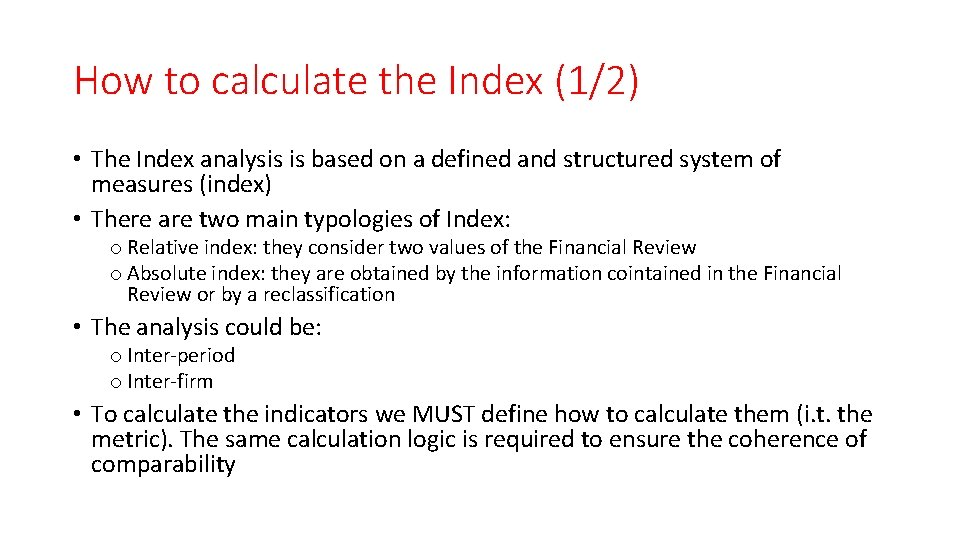How to calculate the Index (1/2) • The Index analysis is based on a defined and structured system of measures (index) • There are two main typologies of Index: o Relative index: they consider two values of the Financial Review o Absolute index: they are obtained by the information cointained in the Financial Review or by a reclassification • The analysis could be: o Inter-period o Inter-firm • To calculate the indicators we MUST define how to calculate them (i. t. the metric). The same calculation logic is required to ensure the coherence of comparability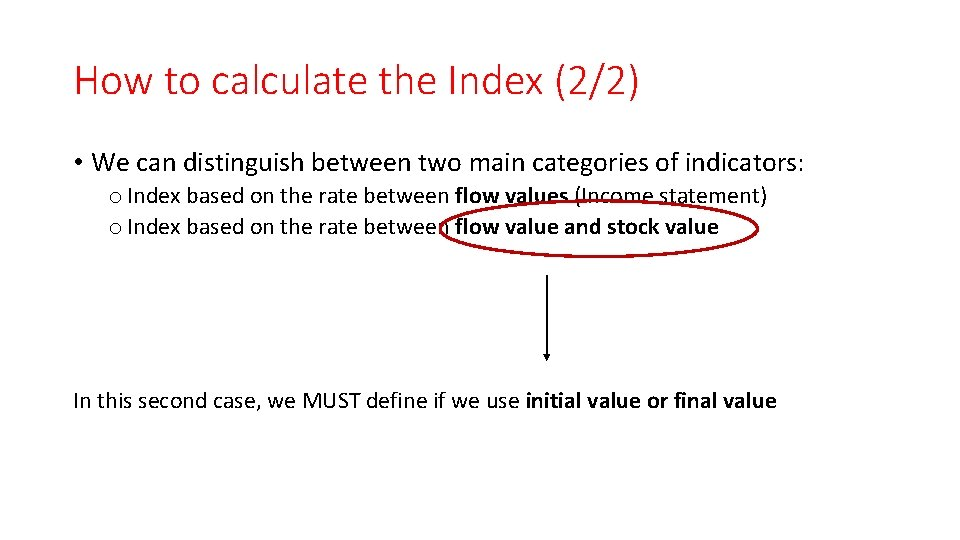How to calculate the Index (2/2) • We can distinguish between two main categories of indicators: o Index based on the rate between flow values (Income statement) o Index based on the rate between flow value and stock value In this second case, we MUST define if we use initial value or final valuePreliminary remarks • Comparability problems arise: o The Income Statement could be drawn up by nature or by function o Different levels of details in the items of the Income Statement Notes to the Financial Statement as a fundamental tool to support the analysis. In fact: – Comparability is ensured by the presence of additional information – If additional information are not available, it is possibile to identify the «non-comparability» areasRatio Analysis limitations • Ratios are presented on a percentage basis • Relative size is ignored • It is assumed that all numbers used are correct • If the numbers are not reliable, ratios are not particularly usefullPROFITABILITY ANALYSIS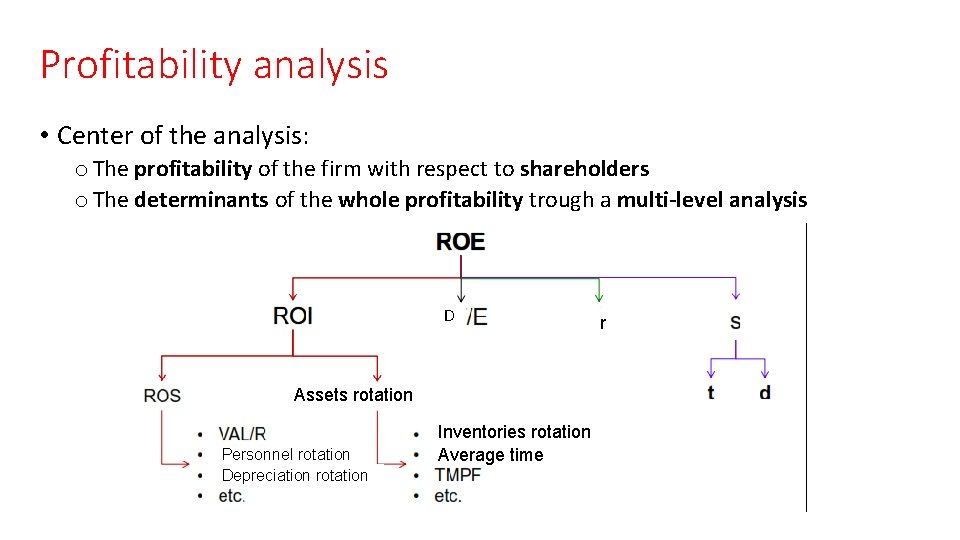Profitability analysis • Center of the analysis: o The profitability of the firm with respect to shareholders o The determinants of the whole profitability trough a multi-level analysis D Assets rotation Personnel rotation Depreciation rotation Inventories rotation Average time r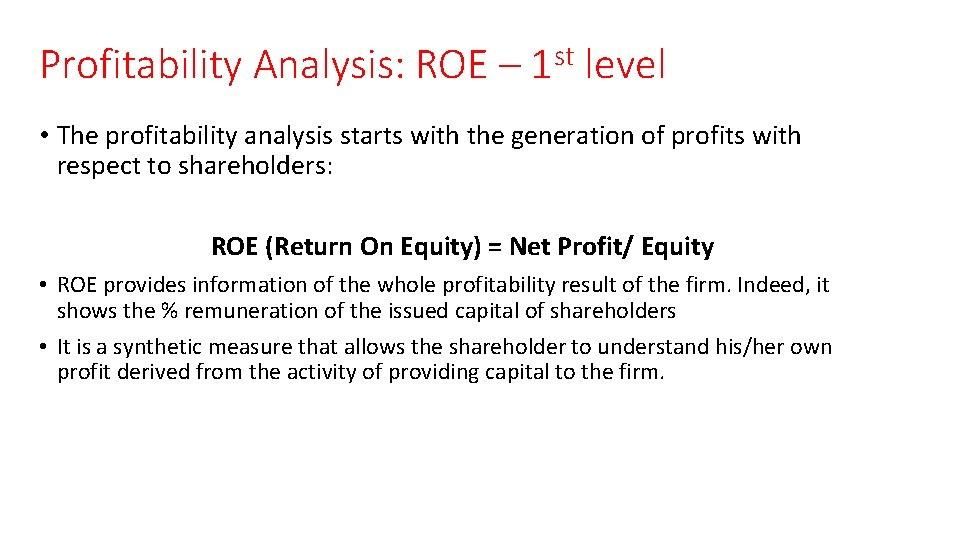Profitability Analysis: ROE – 1 st level • The profitability analysis starts with the generation of profits with respect to shareholders: ROE (Return On Equity) = Net Profit/ Equity • ROE provides information of the whole profitability result of the firm. Indeed, it shows the % remuneration of the issued capital of shareholders • It is a synthetic measure that allows the shareholder to understand his/her own profit derived from the activity of providing capital to the firm.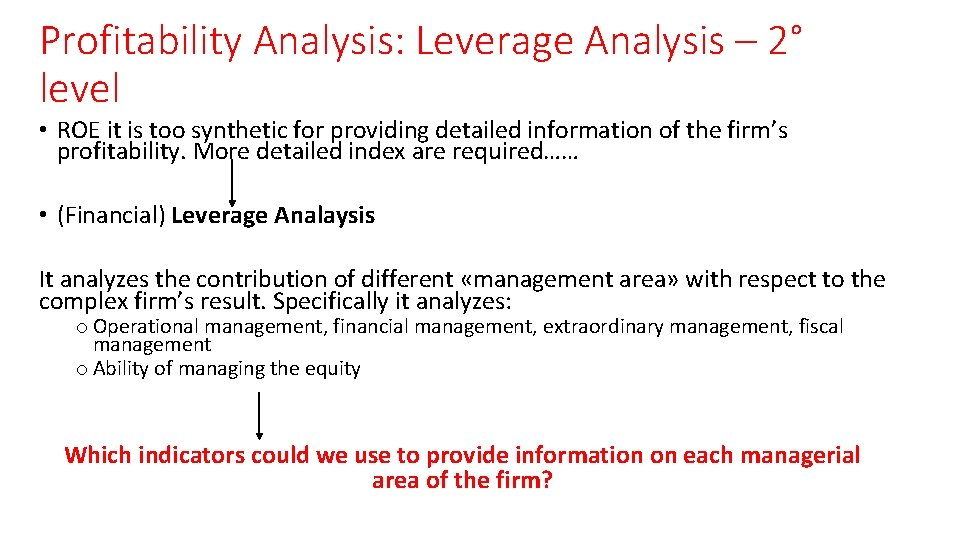Profitability Analysis: Leverage Analysis – 2° level • ROE it is too synthetic for providing detailed information of the firm’s profitability. More detailed index are required…… • (Financial) Leverage Analaysis It analyzes the contribution of different «management area» with respect to the complex firm’s result. Specifically it analyzes: o Operational management, financial management, extraordinary management, fiscal management o Ability of managing the equity Which indicators could we use to provide information on each managerial area of the firm?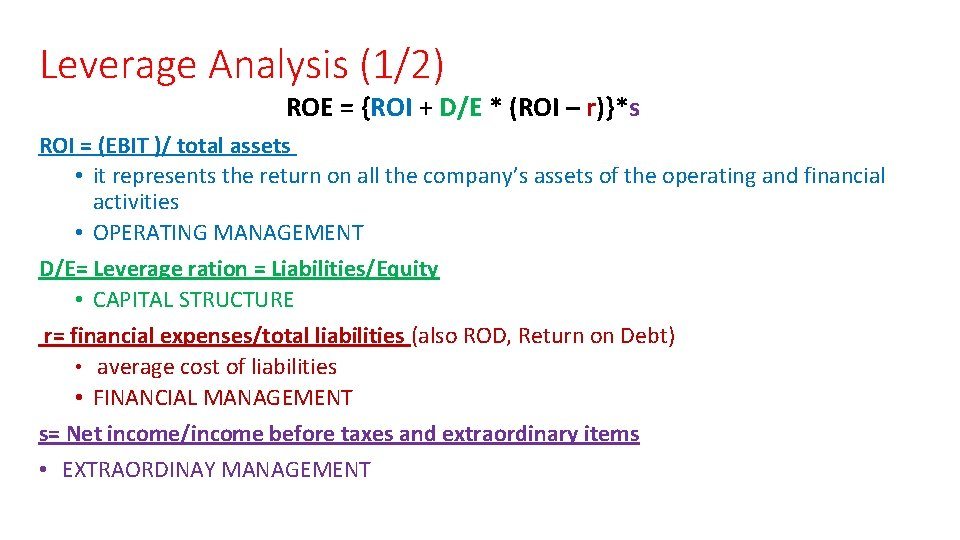Leverage Analysis (1/2) ROE = {ROI + D/E * (ROI – r)}*s ROI = (EBIT )/ total assets • it represents the return on all the company’s assets of the operating and financial activities • OPERATING MANAGEMENT D/E= Leverage ration = Liabilities/Equity • CAPITAL STRUCTURE r= financial expenses/total liabilities (also ROD, Return on Debt) • average cost of liabilities • FINANCIAL MANAGEMENT s= Net income/income before taxes and extraordinary items • EXTRAORDINAY MANAGEMENT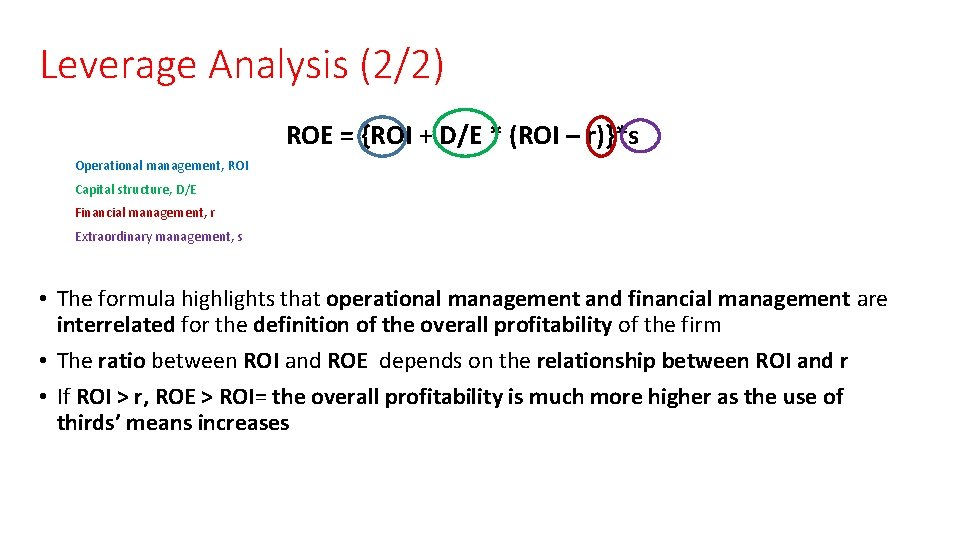Leverage Analysis (2/2) ROE = {ROI + D/E * (ROI – r)}*s Operational management, ROI Capital structure, D/E Financial management, r Extraordinary management, s • The formula highlights that operational management and financial management are interrelated for the definition of the overall profitability of the firm • The ratio between ROI and ROE depends on the relationship between ROI and r • If ROI > r, ROE > ROI= the overall profitability is much more higher as the use of thirds’ means increasesLeverage Analysis (2/2) • It is not worthwhile to increase over a certain level the level of indebtedness (D/E), D/E= levarage ratio = Liabilities/Equity o As the level of indebtedness increases, it is more likely that the average costs asked by third parties to finance the firm increases (= the perceived business risk increases) o ROI is a variable unpredictable and not stocastically defined Equal variations of the profitability level related to the operational management (ROI) or of the cost of thirds’ capital will result, with a higher impact, as higher is the ration leverage/indebtedness. And the business risk increasesProfitability Analysis: ROI Operational Management o ROI (Return on Investment) = Operating profit (E. B. I. T. )/ tot. Assets Income Statement Revenues + Other Revenues + Changes in inventories and WIP -Use/Consumption of raw materials -cost of personnel +/- depriaction and variation of value of non current activities - Other operating costs OPERATING PROFIT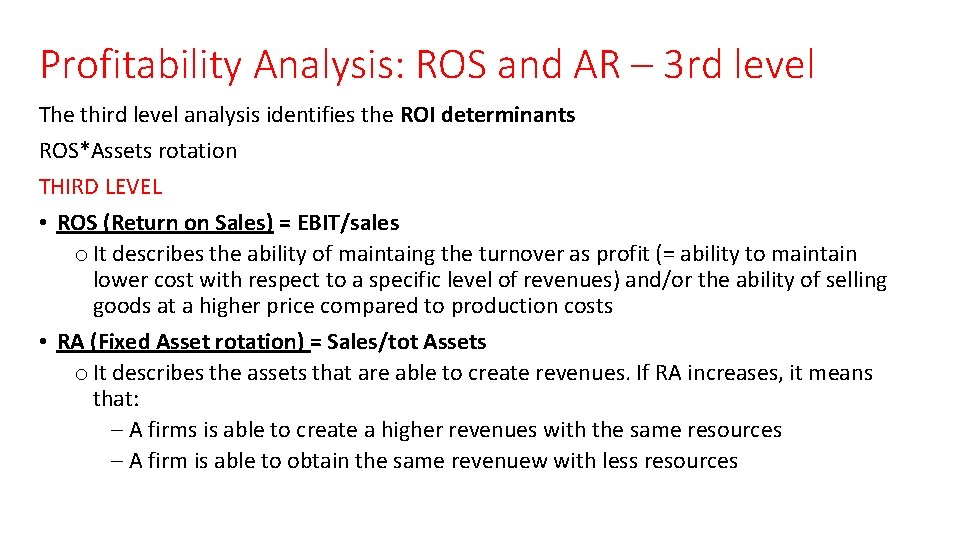Profitability Analysis: ROS and AR – 3 rd level The third level analysis identifies the ROI determinants ROS*Assets rotation THIRD LEVEL • ROS (Return on Sales) = EBIT/sales o It describes the ability of maintaing the turnover as profit (= ability to maintain lower cost with respect to a specific level of revenues) and/or the ability of selling goods at a higher price compared to production costs • RA (Fixed Asset rotation) = Sales/tot Assets o It describes the assets that are able to create revenues. If RA increases, it means that: – A firms is able to create a higher revenues with the same resources – A firm is able to obtain the same revenuew with less resources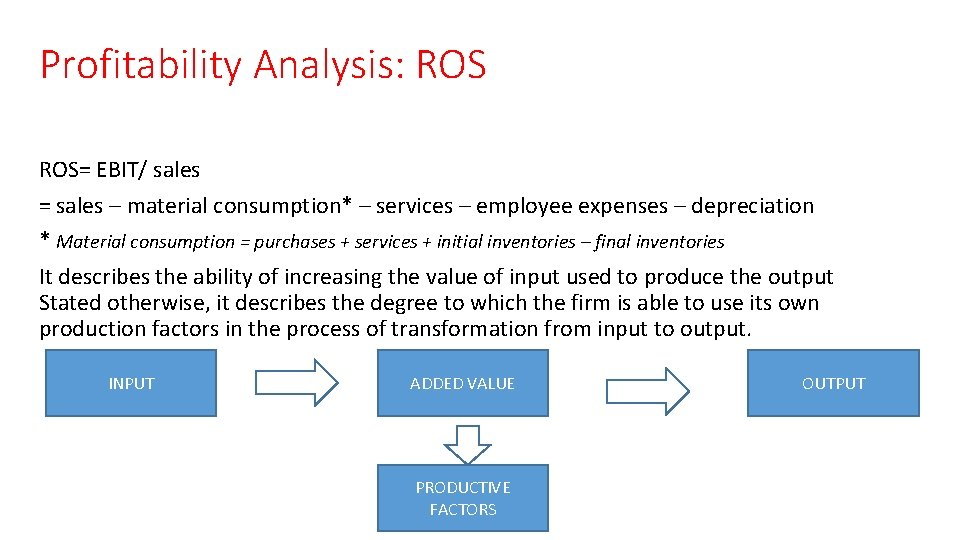Profitability Analysis: ROS= EBIT/ sales = sales – material consumption* – services – employee expenses – depreciation * Material consumption = purchases + services + initial inventories – final inventories It describes the ability of increasing the value of input used to produce the output Stated otherwise, it describes the degree to which the firm is able to use its own production factors in the process of transformation from input to output. INPUT ADDED VALUE PRODUCTIVE FACTORS OUTPUT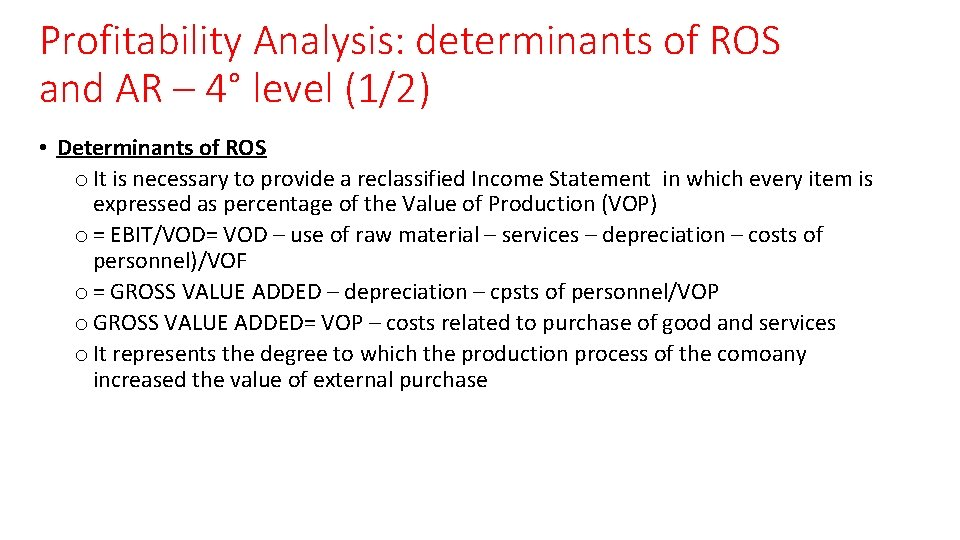Profitability Analysis: determinants of ROS and AR – 4° level (1/2) • Determinants of ROS o It is necessary to provide a reclassified Income Statement in which every item is expressed as percentage of the Value of Production (VOP) o = EBIT/VOD= VOD – use of raw material – services – depreciation – costs of personnel)/VOF o = GROSS VALUE ADDED – depreciation – cpsts of personnel/VOP o GROSS VALUE ADDED= VOP – costs related to purchase of good and services o It represents the degree to which the production process of the comoany increased the value of external purchase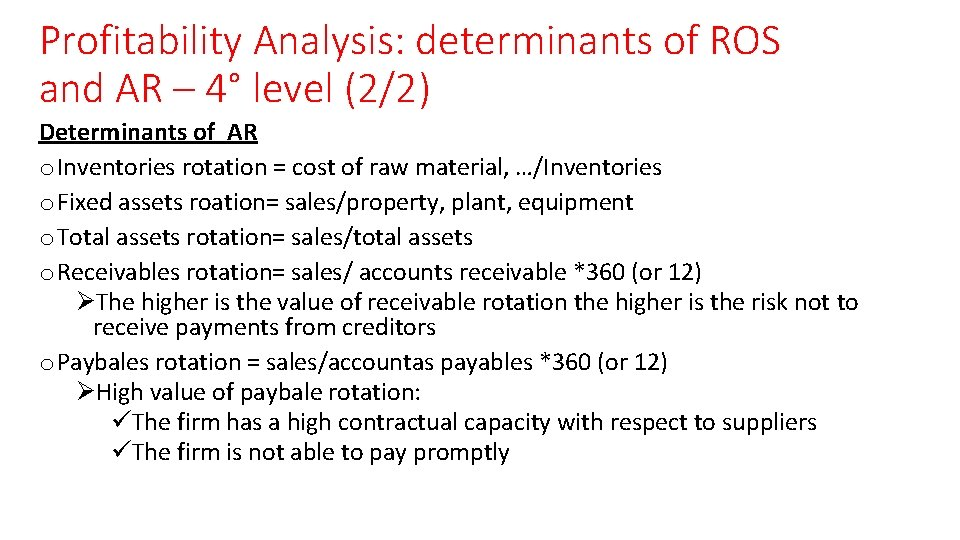Profitability Analysis: determinants of ROS and AR – 4° level (2/2) Determinants of AR o Inventories rotation = cost of raw material, …/Inventories o Fixed assets roation= sales/property, plant, equipment o Total assets rotation= sales/total assets o Receivables rotation= sales/ accounts receivable *360 (or 12) ØThe higher is the value of receivable rotation the higher is the risk not to receive payments from creditors o Paybales rotation = sales/accountas payables *360 (or 12) ØHigh value of paybale rotation: üThe firm has a high contractual capacity with respect to suppliers üThe firm is not able to pay promptlyProfitability Analysis: determinants of ROS and AR – 4° level – receivables rotation Receivables rotation= sales/ accounts receivable *360 • Sales= 6. 000 • Total amount of receivables= 3. 000 • Receivables rotation= 6. 000/3. 000 • Average time to collect receivables: (3. 000/6. 000)*360= 180 days (3. 000/6. 000)*12 = 6 months (2 time/year) If we know the day/month to collect receivable, we could calculate the value of credit: • Days*revenues/360 • Months*revenues/12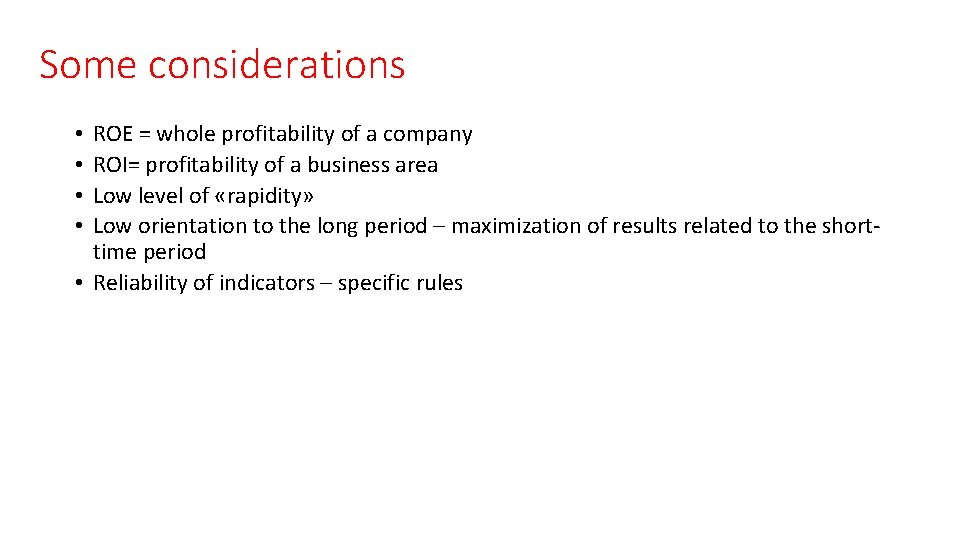Some considerations ROE = whole profitability of a company ROI= profitability of a business area Low level of «rapidity» Low orientation to the long period – maximization of results related to the shorttime period • Reliability of indicators – specific rules • •LIQUIDITY ANALYSIS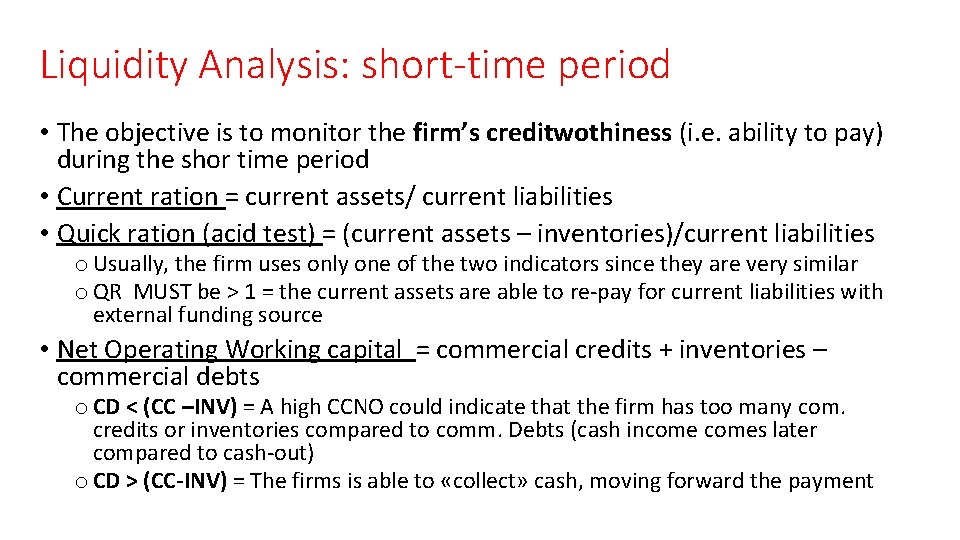Liquidity Analysis: short-time period • The objective is to monitor the firm’s creditwothiness (i. e. ability to pay) during the shor time period • Current ration = current assets/ current liabilities • Quick ration (acid test) = (current assets – inventories)/current liabilities o Usually, the firm uses only one of the two indicators since they are very similar o QR MUST be > 1 = the current assets are able to re-pay for current liabilities with external funding source • Net Operating Working capital = commercial credits + inventories – commercial debts o CD < (CC –INV) = A high CCNO could indicate that the firm has too many com. credits or inventories compared to comm. Debts (cash incomes later compared to cash-out) o CD > (CC-INV) = The firms is able to «collect» cash, moving forward the payment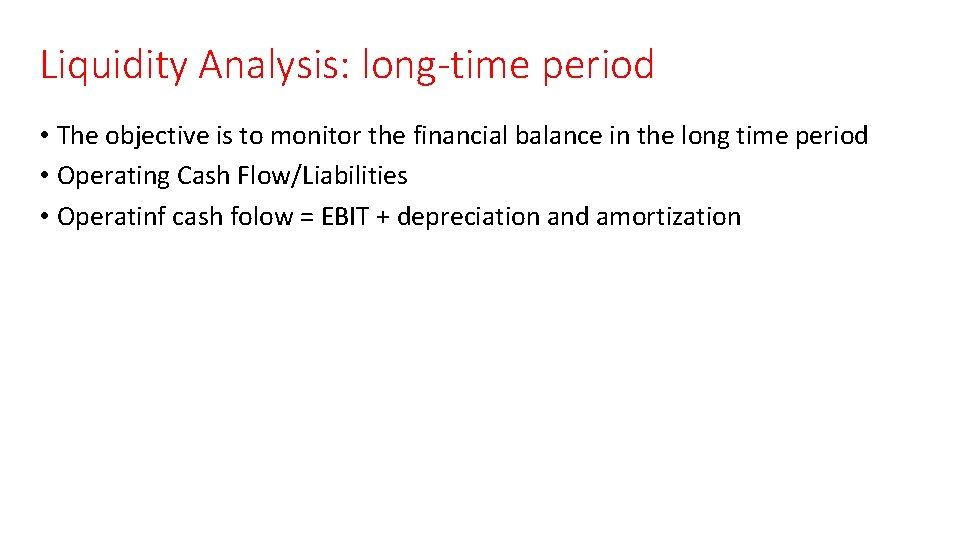Liquidity Analysis: long-time period • The objective is to monitor the financial balance in the long time period • Operating Cash Flow/Liabilities • Operatinf cash folow = EBIT + depreciation and amortization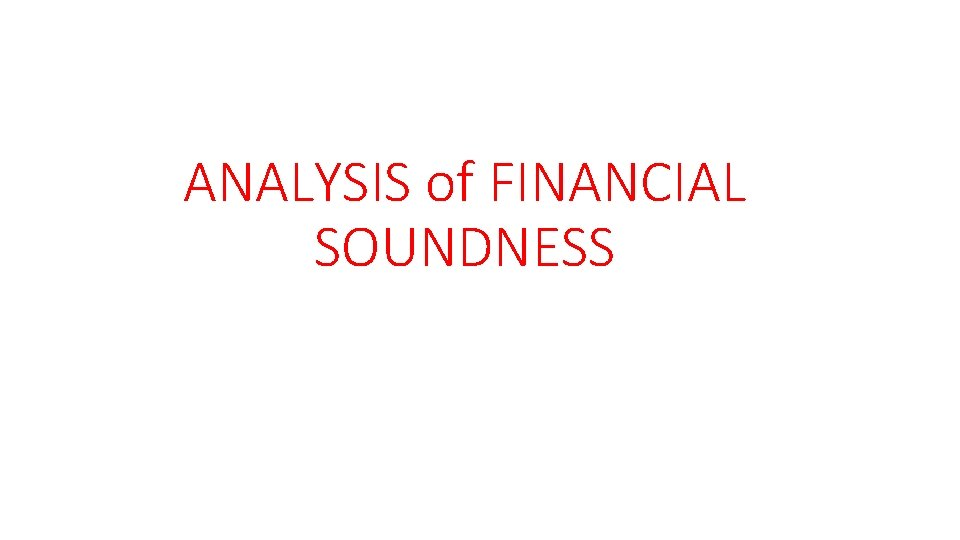ANALYSIS of FINANCIAL SOUNDNESS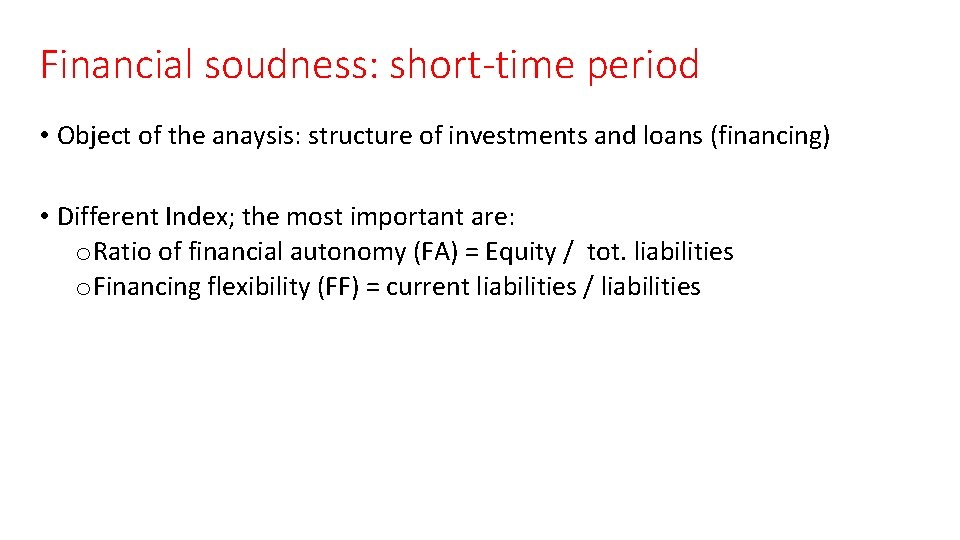Financial soudness: short-time period • Object of the anaysis: structure of investments and loans (financing) • Different Index; the most important are: o Ratio of financial autonomy (FA) = Equity / tot. liabilities o Financing flexibility (FF) = current liabilities / liabilitiesEXERCISE